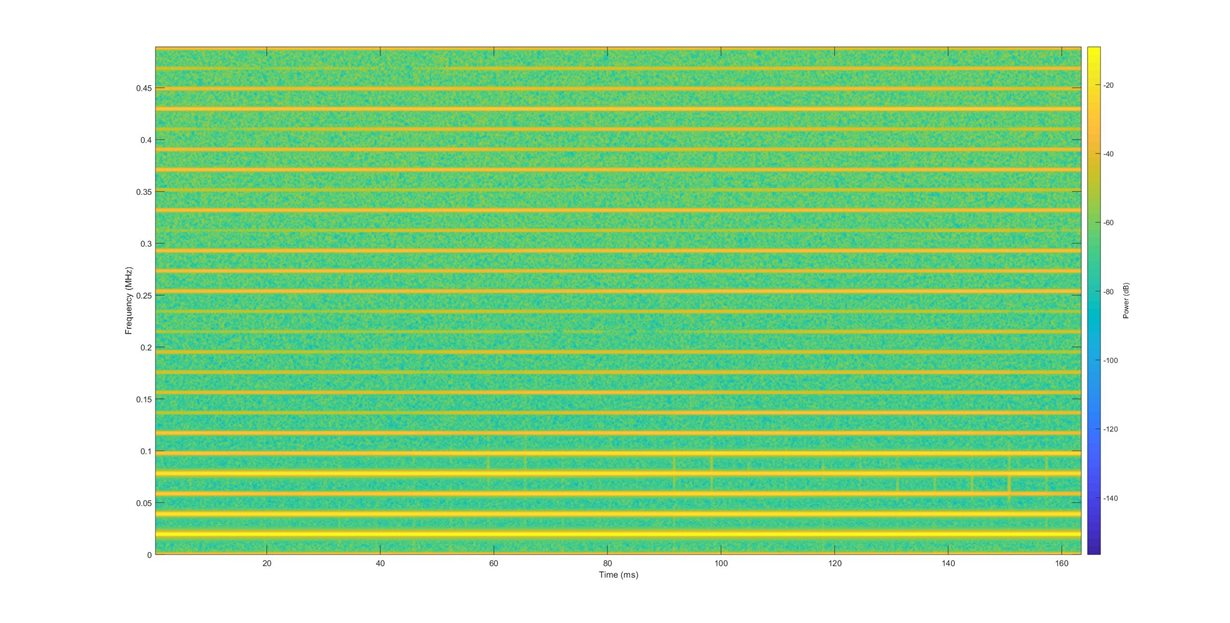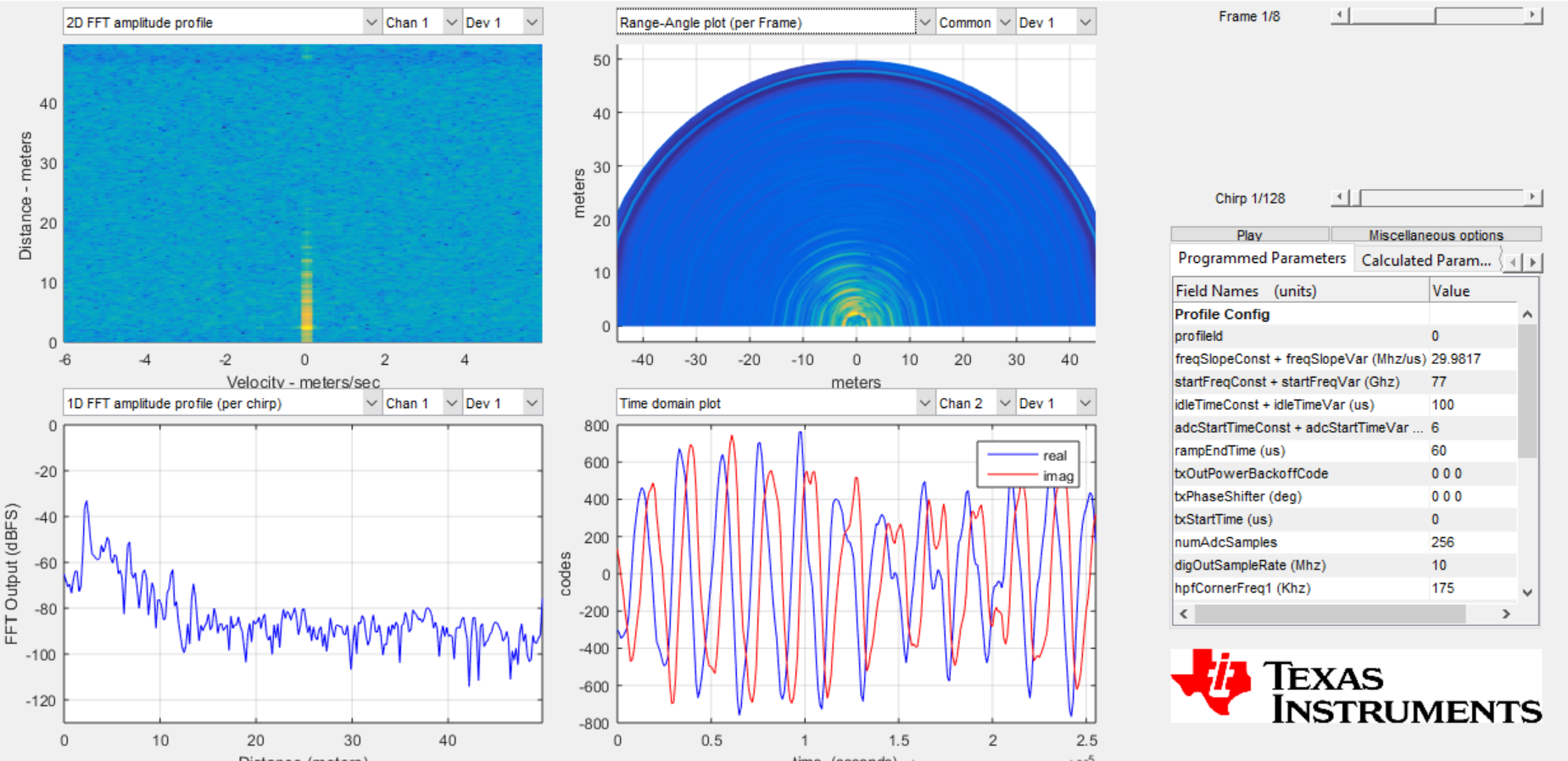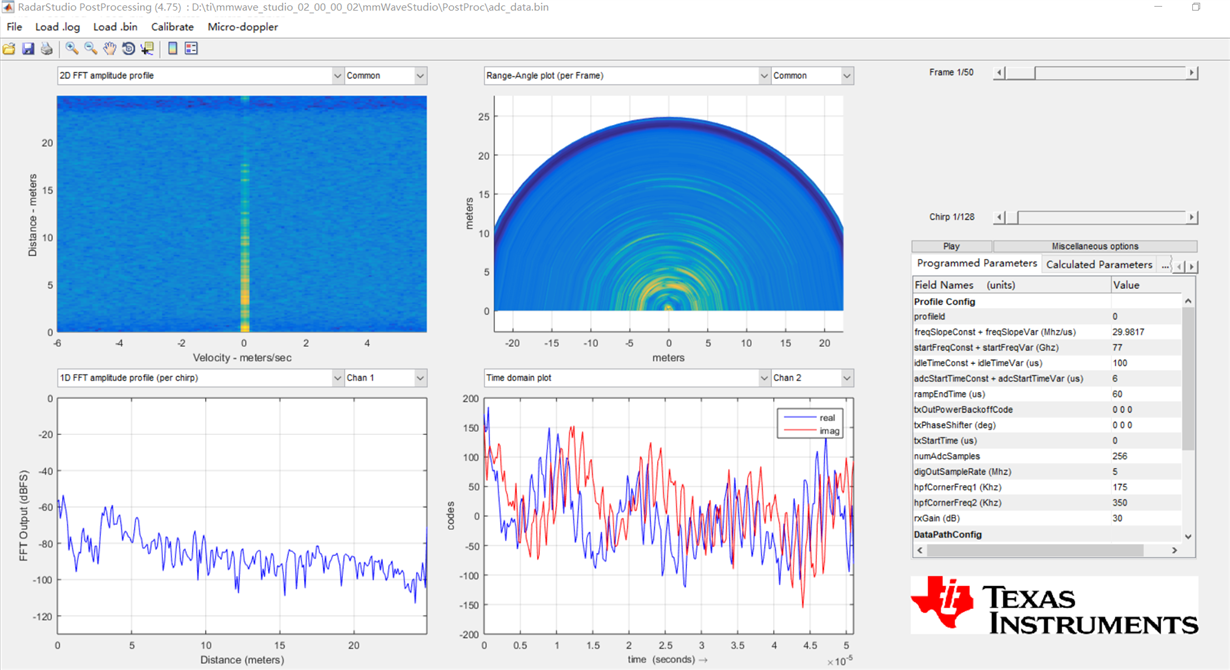If you have a related question, please click the "Ask a related question" button in the top right corner. The newly created question will be automatically linked to this question.

• Resolved

# IWR1642BOOST: Small vibration detection

Part Number: IWR1642BOOST

Hi Team,

Our customer would like to work on human skin vibration detection. He requires a high range resolution (less than 1mm) to detect very small vibrations. Long detection range would also be preferred.

He is looking at IWR1642BOOST but according to him the slope is not enough. Could you recommend a suitable EVM for this? Thanks.

Kind Regards,

Jejomar

• Hi Jejomar,

Those requirements might be too strict for mmWave devices. Can you clarify what you mean by slope? The Sweep Bandwidth is limited to 4GHz by hardware.

Cheers,

Akash

• In reply to Akash Gondalia:

Hello,sir.  I refer the mmwave fundamental pdf, and learn that   f= 2*s*d/c  , in which  the small change in d will have a great effect on f   if there is a big s (which means the slope of the FMCW). Isn' t  it?

1.  The belowing is the time-frequency spectrogram of ADC data (captured by DCA1000).  I want to extract small displacement change in d, which corresponding to a specific frequency. But the resolution of frequency is not enough. So I want to find a more appropriate mmwave radar which can support a big value of slope.

2. By the way, I have another question about the time-frequecy spectrogram. Why there are so many periodic stripes on the spectrogram?  If I'm right, I think if there is only one object  before the radar, there should be only one slope on the spectrogram.Hi Chao,

We are still looking into this and should have an answer for you sometime next week.

Cheers,

Akash

Hi, Jejomar:

You are right.   A target at distance d will results in a IF frequency of f = 2* slope *  d / c.   So slope = 50MHz/us, d = 5m, will results in a IF frequency of 1.667MHz.    A skin vibration of 1mm does mean that the skin will be present be two location at a given time.  it does not mean you need to be able to have a range resolution of 1mm.   You can check the phase change over chirps for this target, that will help you detect the skin vibration.

I see the challenge is that skin is a small part of a person and a person is a bigger target and would be a dominant on the received signal.  It is hard to separate the skin part from the result of the body reflection.   I do not know if you have a horn antenna (very narrow field of view) antenna, whether it is possible.

Regarding your figure, does post processing in DCA1000 provide time frequency spectrometer figure?   If not, how did you calculate time frequency spectrometer?

In your figure, when you say you have single target, do you mean you have a corner reflector and very clean environment?

Can you also send out the post processing results as the figure below?• In reply to zigang Yang:

Hi, Zigang Yang:

Thanks for your answers. (I am Chinese. Considering my poor English, I think I can express myself better if we can talk in Chinese .  hahahahahah~) Here is the answer to your questions.

1. Q:Regarding your figure, does post processing in DCA1000 provide time frequency spectrometer figure?   If not, how did you calculate time frequency spectrometer?

A: No. The post processing of mmWave studio does not support time frequency spectrometer. The following is what I do:  I first get the raw data  (50 frames) captured by DCA1000, and use the 256*128*50 samples to plot the time frequency spectrometer figure by matlab (using the function: [s,f,t] = spectrogram(x,window,noverlap,f,fs). x is just the 256*128*50, which is got by concatenating all the chirps.)  (I doubt if such operation is correct or not, because  the chirps are not continious.)

2. Q:In your figure, when you say you have single target, do you mean you have a corner reflector and very clean environment?

A: There is a wall behind me(about 2.6m). I set the IWR1642 on the desk and target my throat region at a distance of 35cm.

3. Q:Can you also send out the post processing results as the figure below?

A:4. Besides, I wonder if we can change the sampling rate and samples (256 as default) without using mmWave Studio , because the maximum of sampling rate and samples are not enough for me (when I increase the samples per chirp, there will be a warning that the sampling rate should be larger. But it seems that with a setup with 512 samples per chirp, the sampling rate has reached the maximum). If any possibility, just increase the samples per chirp is also a good news to me.Here is the MATLAB code to plot the time frequency spectrogram.

```% specgram of mmwave data
clc;
clear;

numsample = 256;
numchirp = 128;
numframe = 125;
numframe = 50;
perframe = numsample*numchirp;
total_sample = numsample * numchirp * numframe;

max_value = max(abs(input(2,:)));

y = input(2,1:256);
N= 256;
window_type = hanning(N);
nfft = 4096;              % freq-resolution increases with growing of nfft, but with lower instint-power resolution
noverlap = N*31/32;       % time-resolution increase with growing of noverlap( < nfft )
fs = 5000000;
thresh = -60;

spectrogram(y,window_type,noverlap,nfft,fs,'power','yaxis');

```

Hi,

For your spectrogram, it is hard for me to help you since I do not know whether your readDCA1000 function is correct or not.  Maybe you can do a range FFT, and plot the range FFT output, and check whether it looks the same as the one in the post processing window (the bottom-left figure).

Your post processing attachment looks OK to me that we do see a peak at very close in range.

Regarding your questions on (4), you need to increase the ramp end time if you want to increase the number of ADC samples in your profile configuration.  You can also use sensor estimator to design your chirp: https://dev.ti.com/gallery/view/1792614/mmWaveSensingEstimator/ver/1.3.0/

Best,

Zigang

• In reply to zigang Yang:

Hi, there: